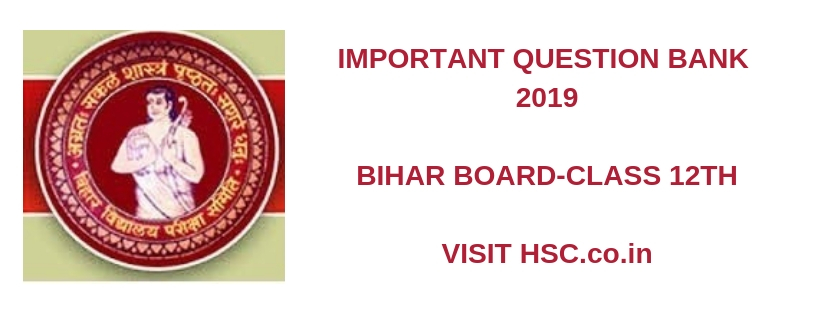# Physics  – Important Question Bank for Bihar Class 12th Intermediate (HSC) Board Exam 2019

HSC Board Exams are fast approaching and students are getting anxious about how to prepare for their HSC Board Exams. So we had mentioned some HSC Study Tips to help students in Cracking HSC Exams.

After the tremendous success of our last year Important Questions Bank for Bihar Class 12 Intermediate (HSC) Board Exam 2016,  2017 and 2018 we have also created a list of Most Important Questions Bank for  Bihar Class 12 Intermediate (HSC) Board Exam 2019 which are likely to appear in HSC Board Exams this year.To unlock the content Click on any of 3 buttons available in the box below saying “This content is Locked”. Once you click on the button the content will get unlocked on same page itself. You must click on social media button showing in below box ie Facebook, Twitter or Google Plus to unlock the content.

1. Obtain an expression for the capacitance of a parallel plate capacitor. The capacitors of capacitances C1, C2 and C3 are connected in series
2. The minimum angular magnification of an astronomical telescope is 10 X. The focal length of objective.
3. The critical angle of prism is 300 with air .The angle of prism is 600. Determine the range of angle of incidences for which emergent ray is obtained.
4. Two inductors each of L=10mH, are connected in parallel but are well separated. Evaluate the effective inductance of configuration.
5. Define resistivity and conductivity of a material gives their SI units and temperature dependence. What do you mean by specific resistance?
6. How is wave front and secondary wavelets defined? Verify laws of reflection or laws of refraction on the basis of Huygens’s wave theory.
7. Two 200 gram pith balls are suspended from a point through two strings of equal length 50 cm. When equal charges are given on the balls, they are repelled and gets separated by 4 cm estimate the charge on each ball.
8. What do you understand by terms “average value”, “r.m.s. value”, “virtual value” and maximum value of an alternating current. Find  expression for them
9. Show that the line integral of the electric field is zero along a closed path what does this result show?
10. State and prove Gauss’s theorem is electrostatics.
11. Define electric field intensity at a point and find its value at a point due to an electric pole when point is Axial or equatorial.
12. What is meant by electric dipole and dipole moment? Show that is a uniform electric field a dipole experiences only a torque and no net force. Derive an expression for the torque.
13. State principle of superposition and its importance calculate electrostatic force and a point charge due to different types of continuous charge distribution.
1. What is the easiest method of charging a body? Explain quantisation of change and conservation of change. Why electrostatic is also called Frictional electricity?
2. Why is a potentiometer preferred our a voltmeter to measure emf of a cell? How can a galvanometer be converted into an ammeter of a voltmeter.
3. State Kirchoff’s law and use it to obtain condition of balance of a whetstone bridge.
4. How internal resistance of a cell can be determined using potentiometer. Explain with help of circuit diagram.
5. What is induced emf? Write Faraday laws of electromagnetic induction obtain an expression for the emf induced in a rotating rod of length ‘l’.
6. Define electric field intensity at a point and find its value at a point due to an electric pole when point is Axial or equatorial
7. What is the purpose of a transformer? Explain its construction, types and working. Write expression for secondary to primary voltage in terms of the no. of terms in the two coils.
8. Draw a labeled ray diagram for the formation of image by a Compound microscope. Derive expression for its total magnification, when final image is formed at the near point. Why both objective and eyepiece of a compound microscope must have their short focal lengths?
9. Two 200 gram pith balls are suspended from a point through two strings of equal length 50 cm. When equal charges are given on the balls, they are repelled and get separated by 4 cm estimate the charge on each ball.
10. What is the principle of a capacitor? Derive an expression for energy stored in a capacitor and hence find the energy density.
11. Describe principle, construction and working of Van-de Graff generator. How the leakage of charge is minimized from the generator.
12. Derive an expression for the resistivity of a conductor in terms of number density of free electrons and relaxation time.
13. What are Coherent Sources? Derive mathematical expression for width of interference fringes obtained in Young’s double slit Experiment. With the help of graph, show the variation of intensity pattern in YDSE.
14. Write principal of Wheatstone bridge. Derive a condition for balanced Wheatstone bridge. Why this arrangement can’t be used to measure very low resistance?
1. Obtain an expression for the capacitance of a parallel plate capacitor. The capacitors of capacitances C1, C2 and C3 are connected in parallel.
2. With the help of neat and labelled diagram, explain the principle and Working of a moving coil galvanometer. What is importance of radial magnetic field?

Hi, we’re trying to collate and gather the data and would be updating it here a few days before the exam. Please keep on visiting our website for updates.

Please use the comments box below and post questions that you think are important from your analysis. It would help the HSC community a lot.

Do subscribe to our updates so that you do not miss out on any important information that we push your way.

Don’t forget to read : MUST REMEMBER THINGS on the day of Exam for HSC Students